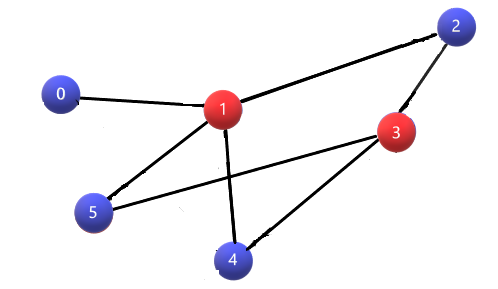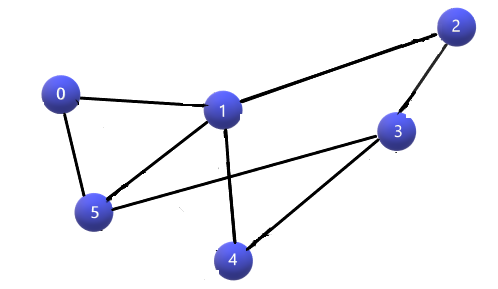# Bipartite Check using DFS – If Graph is Bipartite

Problem Statement: Given is a 2D adjacency list representation of a graph. Check whether the graph is Bipartite graph. (Note: In a Bipartite graph, one can color all the nodes with exactly 2 colors such that no two adjacent nodes have the same color)

Examples:

```Example 1:

Input:

Graph= {
0 -> 1
1 -> 0, 2, 4, 5
2 -> 1, 3
3 -> 2, 4, 5
4 -> 1, 3
5 -> 1, 3
}

Output: It is a Bipartite Graph.

Explanation:
The input graph is basically represented by an adjacency list. Each index denotes a vertex in the graph and also has a list of vertices, with which the index vertex is connected. Here we can see that the nodes can be colored such that the Bipartite graph condition holds. For instance, 0 –blue, 1 – red, 2 - blue, 3 – red, 4 – blue, 5 – blue. Refer to the picture.```
```Example 2:

Input:
Graph= {
0 -> 1, 5
1 -> 0, 2, 4, 5
2 -> 1, 3
3 -> 2, 4, 5
4 -> 1, 3
5 -> 0, 1, 3
}

Output: It is not a Bipartite Graph.

Explanation: This graph is the same as Example1, the difference is, we have one more edge from node 0 to node 5. We can see there are 3 cycles in this graph. Because of cycle 0 -> 1 -> 5 -> 0, it is not a bipartite graph. Let’s see the reason in some time.```

### Solution

Disclaimer: Don’t jump directly to the solution, try it out yourself first.

Intuition: If we look closely, we can notice that if the graph has no cycle, then such a graph is always bipartite(because you can just change the color of neighbour nodes recursively, the bipartite condition still holds). Also, think of this one, in order to prove that the graph is not a Bipartite one, we need to have adjacent nodes with the same color. Since the graph is not going to have a cycle, we still have the opportunity to change the color. So, the question is how to approach graphs with cycles. Let’s see.

Approach:

• In the above examples, we can see that both the graphs have cycles, but the 1st graph is bipartite. How is it possible? Let’s take a closer look at a cycle. From the 2nd example, 0 -> 1 -> 5 -> 0 is a cycle. If 0 is colored with red then 1 and 5 have to be colored with blue. But 1 and 5 are connected. Let’s take the 1st example, 1 -> 2 -> 3 -> 5 -> 1. As usual, 1 – red, 2 – blue, 3 – red, 5 – blue.
• The key point is that if a cycle has an even number of nodes, then there is no issue. But on the other hand, if it has an odd number of nodes, then the Bipartite condition fails. Because we only have 2 colors, if we have an odd number of nodes, definitely the starting and ending nodes will have the same color, but for even it won’t be.
• Our color array basically has 3 states. It tells whether the node has been colored, if colored then whether it is the 1st color or 2nd one.  In the checkBipartite function, we are initializing the color array with -1(not yet colored). We can use the color array to check whether a node is visited or not (we don’t need a separate visited array).
• Then we are checking whether the node is colored or not. If it is not colored, we are coloring with the opposite color of its neighbor. That is, 1 – color[node], gives 1 if the color[node] is 0 and 0 if the color[node] is 1. This is how we are inverting the color of the adjacencies.

If the current neighbor is already colored, we check its color and the variable, node’s color. We know that they are adjacent nodes, if the colors are the same, we can infer that it is not a Bipartite graph, so returning false, else returning true.

Code:

## C++ Code

``````#include <bits/stdc++.h>
using namespace std;

bool bipartiteDfs(int node, vector<int> adj[], int color[]) {
if(color[it] == -1) {
color[it] = 1 - color[node];
return false;
}
} else if(color[it] == color[node]) return false;
}
return true;
}
bool checkBipartite(vector<int> adj[], int n) {
int color[n];
memset(color, -1, sizeof color);
for(int i = 0;i<n;i++) {
if(color[i] == -1) {
color[i] = 1;
return false;
}
}
}
return true;
}
{
}
int main() {

cout << "It is a Bipartite Graph";
} else {
cout << "It is not a Bipartite Graph";
}
return 0;
}``````

Output: It is a Bipartite Graph

Time Complexity: O(V + E), since in its whole, it is a DFS implementation, V – vertices; E – edges;

Space Complexity: O(V), because, apart from the graph, we maintain a color array.

## Java Code

``````import java.util.*;
class Solution {
static boolean dfsCheck(ArrayList < ArrayList < Integer >> graph, int node, int color[]) {
for (Integer it: graph.get(node)) {
if (color[it] == -1) {
//Color of variable neighbor is the inverted color of variable node
color[it] = 1 - color[node];

if (!dfsCheck(graph, it, color))
return false;
} else if (color[it] == color[node]) {
return false;
}
}
return true;
}

static boolean checkBipartite(ArrayList < ArrayList < Integer >> graph, int n) {
int color[] = new int[n];

for (int i = 0; i < n; i++) {
color[i] = -1;
}

for (int i = 0; i < n; i++) {
if (color[i] == -1) {
if (!dfsCheck(graph, i, color)) {
return false;
}
}
}
return true;
}

public static void main(String args[]) {
int n = 6;
ArrayList < ArrayList < Integer >> graph = new ArrayList < ArrayList < Integer >> ();

for (int i = 0; i < n; i++)
graph.add(new ArrayList < Integer > ());

if (checkBipartite(graph, n))
System.out.println("It is a Bipartite Graph");
else
System.out.println("It is not a Bipartite Graph");
}
}``````

Output: It is a Bipartite Graph

Time Complexity: O(V + E), since in its whole, it is a DFS implementation, V – vertices; E – edges;

Space Complexity: O(V), because, apart from the graph, we maintain a color array.